# 17.2 Programming Cryptographic Keys

In the following sections, we demonstrate how to use the .NET support for creating and managing keys. Some of these techniques are functionality equivalent, and when deciding between them for your projects, you must take into consideration the tension between the abilities of the user and the demands for data security; see Section 17.1.1 for details.

#### 17.2.1 Creating Keys

In the following sections, we discuss three techniques for creating keys. Only one of these techniques presents the user with data that is easy to memorize. You must be pragmatic when deciding how to create new keys, and select a process that satisfies the security demands of your project and the practical demands of the users.

##### 17.2.1.1 Using the algorithm classes

The simplest way to create keys is to use the functionality built into all of the .NET algorithm classes for both symmetric and asymmetric algorithms. The .NET classes creates new keys as they are needed; if you attempt to perform any cryptographic operation and you have not explicitly specified the keys to use, then the .NET classes will create new keys automatically. The following statements demonstrate how to use this functionality to print out the key value for a symmetrical algorithm (for full details see Chapter 14):

`# C# // create an instance of the symmetric algorithm SymmetricAlgorithm x_alg = SymmetricAlgorithm.Create("Rijndael"); // set the length of key that we want to create x_alg.KeySize = 128; // get the key value, which will cause the implementation // class to create a new secret key byte[] x_secret_key = x_alg.Key; // print out the key foreach (byte b in x_secret_key) {     Console.Write("{0:X2} ", b); } # Visual Basic .NET ' create an instance of the symmetric algorithm Dim x_alg As SymmetricAlgorithm = SymmetricAlgorithm.Create("Rijndael") ' set the length of key that we want to create x_alg.KeySize = 128 ' get the key value, which will cause the implementation ' class to create a new secret key Dim x_secret_key As Byte(  ) = x_alg.Key ' print out the key Dim b As Byte For Each b In x_secret_key     Console.Write("{0:X2} ", b) Next b`

The following statements demonstrate how to create a new key and print out the value for an asymmetric algorithm; unlike most asymmetric functions, obtaining details of the key can be performed through the abstract AsymmetricAlgorithm class (for full details see Chapter 15):

`# C# // create an instance of the asymmetric algorithm AsymmetricAlgorithm x_alg = AsymmetricAlgorithm.Create("RSA"); // set the length of key that we want to create x_alg.KeySize = 1024; // get the key value, which will cause the implementation // class to create a new secret key string x_key_pair = x_alg.ToXmlString(true); // print out the key Console.WriteLine(x_key_pair); # Visual Basic .NET ' create an instance of the asymmetric algorithm Dim x_alg As AsymmetricAlgorithm = AsymmetricAlgorithm.Create("RSA") ' set the length of key that we want to create x_alg.KeySize = 1024 ' get the key value, which will cause the implementation ' class to create a new secret key Dim x_key_pair As String = x_alg.ToXmlString(True) ' print out the key Console.WriteLine(x_key_pair)`

The principal benefit of creating keys in this manner is that you do not have to have any prior knowledge about how the key should be created; the algorithm class is responsible for creating keys in accordance with the relevant specification. The principle drawback is that the .NET classes generate keys that users will find difficult to remember.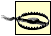When using an algorithm class to create a new key, you must be confident that the implementation creates keys securely and there are no flaws in the implementation that might result in key values that are easily guessed.

##### 17.2.1.2 Using a random number generator

The second approach to creating keys is to use a random number generator (RNG). The .NET Framework provides support classes for generating random data; Figure 17-3 illustrates the class hierarchy.

##### Figure 17-3. The.NET Framework class hierarchy for random number generators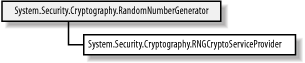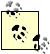You cannot generate random data to create a key pair for an asymmetric algorithm. You must rely on the implementation classes to create key pairs or follow the appropriate key generation protocol; see Chapter 15 for details. Asymmetric algorithms create keys using numbers that are mathematically related and cannot be created from random data.

The class hierarchy for random number generators follows the abstract class/implementation class model that is used for hashing algorithms and symmetrical algorithms. The .NET Framework includes one implementation class, which is a wrapper around the RNG functions of the native Windows Cryptography API. Table 17-1 details the public members of the abstract RandomNumberGenerator class.

##### Table 17-1. Members of the RandomNumberGenerator class

Member

Description

Create

Creates an instance of a RandomNumberGenerator implementation class by name or creates an instance of the default implementation class if no name is specified

GetBytes

Populates a byte array with a sequence of random values

GetNonZeroBytes

Populates a byte array with a sequence of random values, none of which will be 0

You must know how many bytes of random data to create to prepare a key for a given algorithm correctly. The following statements demonstrate how to use the random number generator to create a key for a symmetric algorithm:

`# C# // create an instance of the symmetric algorithm SymmetricAlgorithm x_alg = SymmetricAlgorithm.Create("Rijndael"); // set the length of key that we want to create x_alg.KeySize = 128; // we need to create an array of 16 bytes (16 bytes is 128 bits) byte[] x_key_data = new byte; // create the RNG RandomNumberGenerator x_rng = RandomNumberGenerator.Create(  ); // use the RNG to populate the byte array with random data x_rng.GetBytes(x_key_data); // set the key value for the symmetrical algorithm x_alg.Key = x_key_data; # Visual Basic .NET ' create an instance of the symmetric algorithm Dim x_alg As SymmetricAlgorithm = SymmetricAlgorithm.Create("Rijndael") ' set the length of key that we want to create x_alg.KeySize = 128 ' we need to create an array of 16 bytes (16 bytes is 128 bits) Dim x_key_data(15) As Byte ' create the RNG Dim x_rng As RandomNumberGenerator = RandomNumberGenerator.Create(  ) ' use the RNG to populate the byte array with random data x_rng.GetBytes(x_key_data) ' set the key value for the symmetrical algorithm x_alg.Key = x_key_data`
##### 17.2.1.3 Using a key-derivation protocol

The previous two approaches result in values that a user will find difficult to remember. Users tend to prefer key values that have some meaning, but keys made up of proper words do not make ideal cryptographic keys; they are more susceptible to brute force attacks, because not all key values are equally likely when we are restricted to alphanumeric values listed in a dictionary.

A key-derivation protocol is a compromise between the need to create keys that are difficult to guess and the need for users to remember the key values. This kind of protocol processes a password selected by the user to create a cryptographic key. The user remembers the password, and types it in (rather than a sequence of numeric values). The derivation protocol transforms the password into a cryptographic key. The .NET Framework supports one key-derivation protocol, which is based on the PKCS #5/PBKDF1 standard. We summarize the protocol, as follows:

1. Alice selects a password. The password can be made up of more than one word for example, "Programming .NET Security" would be acceptable as a password; though the word "password" is used, "pass phrase" is more appropriate.

2. Alice generates eight bytes of random data, known as the salt. The salt value is not secret, and Alice can safely write it down. The benefit of adding random data to the password is that two users who select the same password will end up with different cryptographic keys.

3. Alice concatenates her password and the salt data together to form the data block.

4. Alice selects a hashing algorithm and creates a hash code for the data block.

5. For a specified number of iterations, Alice uses the hashing algorithm to create a new hash code, using the previous hash code as the input. The first hash code is produced by hashing the data block. The second hash code is produced by hashing the first hash code. The third hash code is created by hashing the second hash code, and so on. It is common to perform 100 iterations.

6. Alice uses the first n bytes of the final hash code to create a cryptographic key of 8n bits. For example, if Alice selected the SHA-1 hashing algorithm, she will acquire a hash code that is 20 bytes long (because SHA-1 produces 160-bit hash codes). If Alice required a 128-bit key, she will use the first 16 bytes of the hash code value.

Key-derivation protocols are deterministic, meaning that they will always create the same cryptographic key when supplied with specific password and salt values. Keys derived from passwords are suitable for symmetric algorithms, but asymmetric algorithms require you to follow the appropriate key-generation protocol to create new key pairs. Keys that are derived in this way are not as secure as those created from random data, and the password should be chosen so that it is difficult to guess.

Figure 17-4 illustrates the .NET Framework class hierarchy for derivation protocols. The PasswordDerivedBytes class implements the protocol we described; the members of this class are listed in Table 17-2.

##### Figure 17-4. The .NET Framework class hierarchy for byte derivation schemes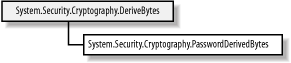##### Table 17-2. PasswordDeriveBytes Members

Member

Description

Properties

HashName

The name of the hashing algorithm to use; see Chapter 13 for details of the supported algorithms

IterationCount

The number of iterations to perform to create the final hash code

Salt

A byte array representing the salt data

Methods

GetBytes

Creates the final hash code and returns a number of bytes, specified as an integer method argument

The following statements demonstrate how to use the PasswordDeriveBytes class to derive a key using "Programming .NET Security" as the password; the password and the salt value are specified in the class constructor:

`# C#         // create the random salt value byte[] x_salt = new byte; RandomNumberGenerator x_rand = RandomNumberGenerator.Create(  ); x_rand.GetBytes(x_salt);          // create the derivation protocol class PasswordDeriveBytes x_pwd      = new PasswordDeriveBytes("Programming .NET Security", x_salt); // specify the number of iterations x_pwd.IterationCount = 100; // specify the hashing algorithm x_pwd.HashName = "SHA1";          // create the key byte[] x_key = x_pwd.GetBytes(16); // write out the salt value Console.Write("SALT: "); foreach (byte b in x_salt) {     Console.Write("{0:X2} ", b); } Console.WriteLine(  );          // write out the key value Console.Write("KEY: " ); foreach (byte b in x_key) {     Console.Write("{0:X2} ", b); } Console.WriteLine(  ); # Visual Basic .NET ' create the random salt value Dim x_salt(7) As Byte Dim x_rand As RandomNumberGenerator = RandomNumberGenerator.Create(  ) x_rand.GetBytes(x_salt) ' create the derivation protocol class Dim x_pwd As PasswordDeriveBytes _ = New PasswordDeriveBytes("Programming .NET Security", x_salt) ' specify the number of iterations x_pwd.IterationCount = 100 ' specify the hashing algorithm x_pwd.HashName = "SHA1" ' create the key Dim x_key(  ) As Byte = x_pwd.GetBytes(16) ' write out the salt value Dim b As Byte Console.Write("SALT: ") For Each b In x_salt     Console.Write("{0:X2} ", b) Next Console.WriteLine(  ) ' write out the key value Console.Write("KEY: ") For Each b In x_key     Console.Write("{0:X2} ", b) Next Console.WriteLine(  )`

#### 17.2.2 Using Key Persistence

The implementation classes for the DSA and RSA algorithms are wrappers around native code contained in the Windows Crypto API. These classes expose a feature of this API that allows asymmetric key pairs to be stored persistently by the operating system; the user does not have to remember the key parameters, which are protected by the Windows account password.

Relying on the security of the Windows operating system to protect cryptographic key pairs is not suitable for all projects; projects that require high levels of security are likely to be affected by the relative ease with which a Windows password can be attacked. The full details of the Windows Crypto API are beyond the scope of this book, but in this section, we briefly demonstrate how to use the .NET classes to store key pairs persistently.

The System.Security.Cryptography.CspParameters class allows you to specify the details of how a key pair should be stored there are various options available, but for your purposes, you require only a name to associate with the keys. The name relates to the key store, and you can use any name that suits your needs; the following statements create an instance of the CspParameters class and set the key store name to be MyKeys:

`# C# // create the parameters instance CspParameters x_params = new CspParameters(  ); // specify the container name x_params.KeyContainerName = "MyKeys"; # Visual Basic .NET ' create the parameters instance Dim x_params As CspParameters = New CspParameters(  ) ' specify the container name x_params.KeyContainerName = "MyKeys"`

Once you have created your CspParameters instance, you can use it as the argument to the constructor of the algorithm implementation class. The final step is to set the PersistKeyInCsp property to true, specifying that you want to store the algorithm's key pair persistently. The following statements demonstrate how to create an instance of the RSACryptoServiceProvider class using the CspParameters instance as an argument and set the PersistKeyInCsp property:

`# C#          // create an instance of the crypto provider class with the csp parameters RSACryptoServiceProvider x_rsa = new RSACryptoServiceProvider(x_params); // enable key persistence x_rsa.PersistKeyInCsp = true; # Visual Basic .NET ' create an instance of the crypto provider class with the csp parameters Dim x_rsa As RSACryptoServiceProvider = New RSACryptoServiceProvider(x_params) ' enable key persistence x_rsa.PersistKeyInCsp = true`

The asymmetric implementation classes will create a key pair automatically before performing any cryptographic operations or exporting the key parameters. When the key pair is created, it will be stored persistently and the user will not have to try and remember (or write down) the key details. This technique can be used only with classes that build on functionality of the Windows Crypto API; implementations from third-party companies may not provide this support.

#### 17.2.3 Key Exchange Formatting

The .NET Framework includes classes specifically for exchanging session keys using asymmetric encryption. The process for encrypting a key value is as follows:

1. Create a new instance of the appropriate symmetric algorithm class and create a new session key.

2. Create a new instance of an asymmetric algorithm class and import the public key that you wish to use to encrypt the session key value.

3. Create a new instance of a key exchange formatter and specify the asymmetric class created in the previous step.

4. Encrypt the session key value using the key exchange formatter.

The formatter class is responsible for preparing the session key data prior to encryption with the asymmetric algorithm. Figure 17-5 illustrates the .NET class hierarchy for key exchange formatter classes. The .NET class library includes formatting classes for the RSA algorithm; the DSA algorithm is not suitable for session key exchange since it does not support data encryption.

##### Figure 17-5. The .NET Framework class hierarchy for key exchange formatting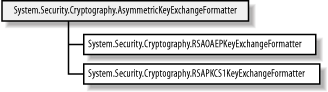Two formatting schemes are supported the OAEP scheme is preferred. The second scheme, PKCS #1 v1.5, is included for compatibility with legacy systems. The formatting scheme is used to protect the session key value from specific attacks; we implement the OAEP scheme in the Section 17.3 of this chapter, but it is not essential that you understand the details of the formatting schemes available, only that you know how to use them. Table 17-3 summarizes the members of the abstract AsymmetricExchangeFormatter class.

##### Table 17-3. AsymmetricExchangeFormatter members

Member

Description

Properties

SetKey

Sets the instance of the asymmetric algorithm implementation class to encrypt the session key

CreateKeyExchange

Encrypts a session key, expressed as an array of bytes

Methods

Parameters

Returns the parameters of the key used to encrypt the session key

Example 17-1 statements demonstrate how to create and format a 128-bit Rijndael session key using the RSA algorithm and the OAEP formatting scheme:

##### Example 17-1. bit Rijndael session key
`# C# // create an instance of the Rijndael algorithm and // specify 128-bits as the key length Rijndael x_rijndael = Rijndael.Create(  ); x_rijndael.KeySize = 128; // rely on the fact that the algorithm implementation // class will create a new key to obtain the session value byte[] x_session_key = x_rijndael.Key; // create an instance of the RSA algorithm class RSA x_rsa = new RSACryptoServiceProvider(  ); // // ... specify public key to encrypt with // // create a new instance of the RSA OAEP formatter RSAOAEPKeyExchangeFormatter x_formatter      = new RSAOAEPKeyExchangeFormatter(  ); // specify the RSA instance we created as the one // to use when encrypting the session key data x_formatter.SetKey(x_rsa); // encrypt the session key with the formatter byte[] x_exchange_data = x_formatter.CreateKeyExchange(x_session_key); // write out the encrypted data foreach (byte b in x_exchange_data) {     Console.Write("{0:X2} ", b); } # Visual Basic .NET ' create an instance of the Rijndael algorithm and ' specify 128-bits as the key length Dim x_rijndael As Rijndael = Rijndael.Create(  ) x_rijndael.KeySize = 128 ' rely on the fact that the algorithm implementation ' class will create a new key to obtain the session value Dim x_session_key(  ) As Byte = x_rijndael.Key ' create an instance of the RSA algorithm class Dim x_rsa As RSA = New RSACryptoServiceProvider(  ) ' ' ... specify public key to encrypt with ' ' create a new instance of the RSA OAEP formatter Dim x_formatter As RSAOAEPKeyExchangeFormatter = New RSAOAEPKeyExchangeFormatter(  ) ' specify the RSA instance we created as the one ' to use when encrypting the session key data x_formatter.SetKey(x_rsa) ' encrypt the session key with the formatter Dim x_exchange_data(  ) As Byte = x_formatter.CreateKeyExchange(x_session_key) ' write out the encrypted data Dim b As Byte For Each b In x_exchange_data     Console.Write("{0:X2} ", b) Next`

The output below shows an example of the formatted exchange data; the data will change each time the statements are executed, because a new session key will have been created at random by the Rijndael class:

`C5 3B 7F 1C 44 B9 DF 3C C6 7F 7E 18 3E E7 F9 15 2E 0C B3 2A EF EC 4C EF 45 1D 51 B8 CC D1 FB C8 7F 8B A8 4F 58 92 76 20 61 13 C1 A2 4E 06 50 9A E2 0E 97 34 8A C7  19 8C 21 59 67 30 3A 57 9A E3 B9 4C 5F 56 4F 10 54 1A 83 5B 45 1A 4F 39 A9 C4 64  C2 11 5C 82 6D E1 A9 F3 BD F3 79 87 EA 13 52 2B EF 5F 71 8B 82 08 F6 9D 57 88 43  42 AE 75 E4 DD B9 BD 52 0F DB CD 86 E7 D2 17 0F 2F DB BF A8`

The output is sent to the person with whom you wish to exchange confidential messages. Notice that the encrypted session key is much longer than the 20 bytes that make up the 128-bit Rijndael session key; this is a result of the padding added to the session key during the formatting process; the encrypted data is always the same length to prevent revealing the length of the session key to eavesdroppers. Figure 17-6 illustrates the hierarchy for the classes that decrypt the exchange data and restore the session key value by removing the formatting.

##### Figure 17-6. The .NET Framework class hierarchy for key exchange deformatting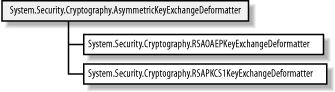The process for decrypting a key value is as follows:

1. Create a new instance of an asymmetric algorithm class and import the private key that you wish to use to decrypt the formatted exchange data.

2. Create a new instance of a key exchange deformatter and specify the asymmetric class created in the previous step.

3. Decrypt the formatted exchange data using the key exchange deformatter.

4. Create a new instance of the appropriate symmetric algorithm class and set the session key.

Table 17-4 summarizes the members of the abstract AsymmetricExchangeDeformatter class.

##### Table 17-4. AsymmetricExchangeDeformatter members

Member

Description

Properties

SetKey

Sets the instance of the asymmetric algorithm implementation class to decrypt the exchange data

DecryptKeyExchange

Decrypts exchange data, expressed as an array of bytes

Methods

Parameters

Returns the parameters of the key used to decrypt the exchange data

The following statements demonstrate how to decrypt the exchange data from the previous example to restore the session key:

`# C# byte[] x_exchange_data = new byte[] {0xC5, 0x3B, 0x7F, 0x1C, 0x44, 0xB9, 0xDF,     0x3C, 0xC6, 0x7F, 0x7E, 0x18, 0x3E, 0xE7, 0xF9, 0x15, 0x2E, 0x0C, 0xB3,      0x2A, 0xEF, 0xEC, 0x4C, 0xEF, 0x45, 0x1D, 0x51, 0xB8, 0xCC, 0xD1, 0xFB,      0xC8, 0x7F, 0x8B, 0xA8, 0x4F, 0x58, 0x92, 0x76, 0x20, 0x61, 0x13, 0xC1,      0xA2, 0x4E, 0x06, 0x50, 0x9A, 0xE2, 0x0E, 0x97, 0x34, 0x8A, 0xC7, 0x19,      0x8C, 0x21, 0x59, 0x67, 0x30, 0x3A, 0x57, 0x9A, 0xE3, 0xB9, 0x4C, 0x5F,      0x56, 0x4F, 0x10, 0x54, 0x1A, 0x83, 0x5B, 0x45, 0x1A, 0x4F, 0x39, 0xA9,      0xC4, 0x64, 0xC2, 0x11, 0x5C, 0x82, 0x6D, 0xE1, 0xA9, 0xF3, 0xBD, 0xF3,      0x79, 0x87, 0xEA, 0x13, 0x52, 0x2B, 0xEF, 0x5F, 0x71, 0x8B, 0x82, 0x08,      0xF6, 0x9D, 0x57, 0x88, 0x43, 0x42, 0xAE, 0x75, 0xE4, 0xDD, 0xB9, 0xBD,      0x52, 0x0F, 0xDB, 0xCD, 0x86, 0xE7, 0xD2, 0x17, 0x0F, 0x2F, 0xDB, 0xBF,      0xA8}; // create an instance of the RSA algorithm class RSA x_rsa = new RSACryptoServiceProvider(  ); // // ... specify private key to decrypt with // // create a new instance of the RSA OAEP deformatter RSAOAEPKeyExchangeDeformatter x_deformatter      = new RSAOAEPKeyExchangeDeformatter(  ); // specify the RSA instance we created as the one // to use when encrypting the session key data x_deformatter.SetKey(x_rsa); // decrypt the exchange data with the deformatter to // obtain the Rijndael session key byte[] x_session_key = x_deformatter.DecryptKeyExchange(x_exchange_data); // create an instance of the Rijndael algorithm Rijndael x_rijndael = Rijndael.Create(  ); // set the session key x_rijndael.Key = x_session_key; # Visual Basic .NET Dim x_exchange_data(  ) As Byte = New Byte(  ) {&HC5, &H3B, &H7F, &H1C, &H44, &HB9, _ &HDF, &H3C, &HC6, &H7F, &H7E, &H18, &H3E, &HE7, &HF9, &H15, &H2E, &HC, &HB3, _ &H2A, &HEF, &HEC, &H4C, &HEF, &H45, &H1D, &H51, &HB8, &HCC, &HD1, &HFB, _ &HC8, &H7F, &H8B, &HA8, &H4F, &H58, &H92, &H76, &H20, &H61, &H13, &HC1, _ &HA2, &H4E, &H6, &H50, &H9A, &HE2, &HE, &H97, &H34, &H8A, &HC7, &H19, _ &H8C, &H21, &H59, &H67, &H30, &H3A, &H57, &H9A, &HE3, &HB9, &H4C, &H5F, _ &H56, &H4F, &H10, &H54, &H1A, &H83, &H5B, &H45, &H1A, &H4F, &H39, &HA9, _ &HC4, &H64, &HC2, &H11, &H5C, &H82, &H6D, &HE1, &HA9, &HF3, &HBD, &HF3, _ &H79, &H87, &HEA, &H13, &H52, &H2B, &HEF, &H5F, &H71, &H8B, &H82, &H8, _ &HF6, &H9D, &H57, &H88, &H43, &H42, &HAE, &H75, &HE4, &HDD, &HB9, &HBD, _ &H52, &HF, &HDB, &HCD, &H86, &HE7, &HD2, &H17, &HF, &H2F, &HDB, &HBF, _ &HA8} ' create an instance of the RSA algorithm class Dim x_rsa As RSA = New RSACryptoServiceProvider(  ) ' ' ... specify private key to decrypt with ' ' create a new instance of the RSA OAEP deformatter Dim x_deformatter As RSAOAEPKeyExchangeDeformatter _ = New RSAOAEPKeyExchangeDeformatter(  ) ' specify the RSA instance we created as the one ' to use when encrypting the session key data x_deformatter.SetKey(x_rsa) ' decrypt the exchange data with the deformatter to ' obtain the Rijndael session key Dim x_session_key(  ) As Byte = x_deformatter.DecryptKeyExchange(x_exchange_data) ' create an instance of the Rijndael algorithm Dim x_rijndael As Rijndael = Rijndael.Create(  ) ' set the session key x_rijndael.Key = x_session_key`

In Chapter 15, we demonstrated that the RSA implementation class always prepares data by applying either OAEP or PKCS #1 v1.5 formatting, making its functionality equivalent to using the formatting classes discussed in this section. These classes define a generic approach to key exchange that can be applied to all implementation classes, including those provided by third parties. In the next section, we define the formatting classes for our ElGamal implementation, which does not format data during encryption automatically.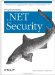Programming .Net Security
ISBN: 0596004427
EAN: 2147483647
Year: 2005
Pages: 346

Similar book on Amazon

flylib.com © 2008-2017.
If you may any questions please contact us: flylib@qtcs.net